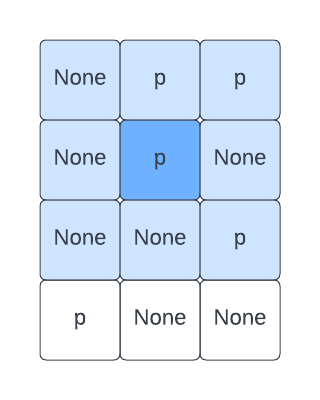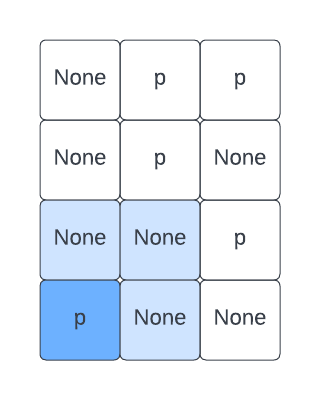Computer Science

# Spatial Logic

Remember the happy puppies problem? A puppy is happy if there is another puppy next to it (up, down, left, right, diagonally):

None, 'p', 'p'
None, 'p', None
None, None, 'p'
'p', None, None

We wrote code to check all 8 directions and it was messy:

def is_happy(grid, row, column):
num_rows = len(grid)
num_columns = len(grid)

# up
if row - 1 >= 0 and is_puppy(grid, row - 1, column):
return True
# down
if row + 1 < num_rows and is_puppy(grid, row + 1, column):
return True
# left
if column - 1 >= 0 and is_puppy(grid, row, column - 1):
return True
# right
if column + 1 < num_columns and is_puppy(grid, row, column + 1):
return True

# diagonal up left
if row - 1 >= 0 and column - 1 >= 0 and is_puppy(grid, row - 1, column - 1):
return True
# diagonal down left
if row + 1 < num_rows and column - 1 >= 0 and is_puppy(grid, row + 1, column - 1):
return True
# diagonal up right
if row - 1 >= 0 and column + 1 < num_columns and is_puppy(grid, row - 1, column + 1):
return True
# diagonal down right
if row + 1 < num_rows and column + 1 < num_columns and is_puppy(grid, row + 1, column + 1):
return True

return False

## Using ranges

Let’s rethink this problem. Here is the case where we check a puppy in the middle of the grid:The puppy we are checking is in dark blue, and the puppies we need to check are in all the surrounding squares, in light blue. Given the (row, column) of a puppy, we can check all of the space around him with:

def is_happy(grid, row, column):
for r in range(row - 1, row + 2):
for c in range(column - 1, column + 2):
# middle position doesn't count, because that is our puppy
if r == row and c == column:
continue
if is_puppy(grid, r, c):
return True

return False

We loop through every possible row from row - 1 to row + 2. We need + 2 because range is not inclusive for the upper end. We likewise loop through every possible column from column - 1 to column + 2. We have to be sure to skip the middle, because that is our puppy and he can’t be happy with just himself.

If we find a puppy anywhere in this range (except the middle), then we return True.

But what about when the puppy is near the edge?Here the puppy to check is at the bottom left. We need to be sure not to check the squares to its left and below it, because those are outside the grid.

We can do this by limiting the range:

def is_happy(grid, row, column):

# setup number of rows and columns
num_rows = len(grid)
num_columns = len(grid)

# find edges of the range for rows
left = max(0, row - 1)
right = min(row + 2, num_rows)

# find edges of the range for columns
up = max(0, column - 1)
down = min(column + 2, num_columns)

# check the area
for r in range(left, right):
for c in range(up, down):
# middle position doesn't count, because that is our puppy
if r == row and c == column:
continue
if is_puppy(grid, r, c):
return True

return False

We can combine this with our is_puppy() function:

def is_puppy(grid, row, column):
if grid[row][column] == 'p':
return True

return False

and our find_happy() function:

def find_happy(grid):
happy = []
for row in range(len(grid)):
for column in range(len(grid[row])):
if is_puppy(grid, row, column) and is_happy(grid, row, column):
happy.append((row, column))
return happy

We can run this with:

grid = [
[None, 'p', 'p'],
[None, 'p', None],
[None, None, 'p'],
['p', None, None],
]
print(find_happy(grid))

and we will get:

[(0, 1), (0, 2), (1, 1), (2, 2)]

## Using ranges and a guard

There is a different way to solve this problem! Instead of restricting our range, let’s always check all the positions around a puppy:

def is_happy(grid, row, column):
for r in range(row - 1, row + 2):
for c in range(column - 1, column + 2):
# middle position doesn't count, because that is our puppy
if r == row and c == column:
continue
if is_puppy(grid, r, c):
return True

return False

and then let’s build a guard into our is_puppy() function:

def is_puppy(grid, row, column):
num_rows = len(grid)
num_columns = len(grid)

# check if the row is off the grid
if row < 0 or row >= num_rows:
return False

# check if the column is off the grid
if column < 0 or column >= num_columns:
return False

if grid[row][column] == 'p':
return True

return False

We can return False any time we are off the grid — no puppy can possibly be there!

Replace the is_happy() functions and is_puppy() functions as shown above, and the code still works.

## Checking larger ranges

Let’s imagine that a puppy is happy if there is a puppy within 3 cells, in any direction. After all, puppies are pretty good at running and finding each other.

We could “hard code” the number 3 into our is_happy() function. But instead, we can use a keyword argument:

def is_happy(grid, row, column, distance=1):
for r in range(row - distance, row + distance + 1):
for c in range(column - distance, column + distance + 1):
# middle position doesn't count, because that is our puppy
if r == row and c == column:
continue
if is_puppy(grid, r, c):
return True

return False

If we use this new version of is_happy() and re-run our code, we will get the same result, because the default distance is 1. But we can change find_happy() as follows:

def find_happy(grid):
happy = []
for row in range(len(grid)):
for column in range(len(grid[row])):
if is_puppy(grid, row, column) and is_happy(grid, row, column, distance=3):
happy.append((row, column))
return happy

We put a 3 in for the distance. Now if we re-run the code, we get:

[(0, 1), (0, 2), (1, 1), (2, 2), (3, 0)]

All of the puppies are happy!